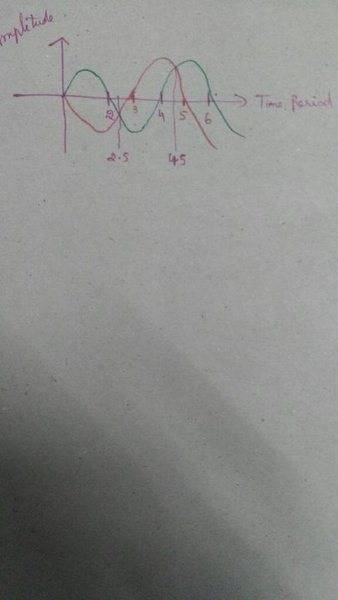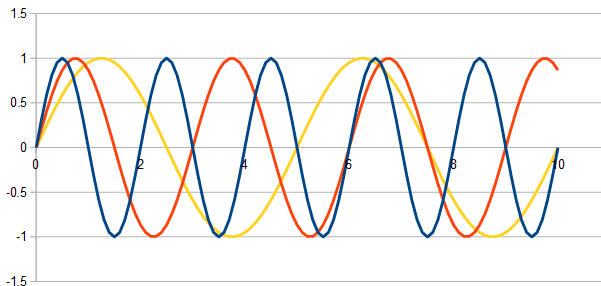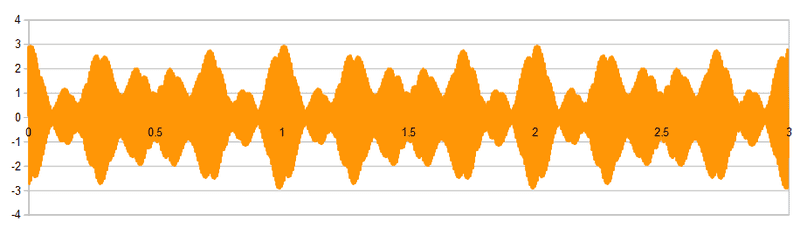# A question about wave motion and beat frequency

• harini07

## Homework Statement

3 tuning forks of frequencies 200, 203, 207 Hz are sounded together.find out the beat frequency.

## Homework Equations

Beat frequency= n1-n2 (n=frequency).

## The Attempt at a Solution

I know that beat frequency is the difference in the frequencies of two superposing notes. But here 3 wave frequencies are given the difference between the first 2 is 3 while between 203, 207 is 4 .How to find the beat frequency of all 3 waves together? I have even tried finding beat frequency of the lowest and highest frequency and got 7 but still it's not the answer.how to solve these kinds sum any little help will be greatly helpful :)

## Homework Statement

3 tuning forks of frequencies 200, 203, 207 Hz are sounded together.find out the beat frequency.

## Homework Equations

Beat frequency= n1-n2 (n=frequency).

## The Attempt at a Solution

I know that beat frequency is the difference in the frequencies of two superposing notes. But here 3 wave frequencies are given the difference between the first 2 is 3 while between 203, 207 is 4 .How to find the beat frequency of all 3 waves together? I have even tried finding beat frequency of the lowest and highest frequency and got 7 but still it's not the answer.how to solve these kinds sum any little help will be greatly helpful :)
You need to start with a general concept of what a beat frequency is. How would you define it?

Beats per second.i.e. difference between 2 interfering waves' frequency

Beats per second.i.e. difference between 2 interfering waves' frequency
No, that doesn't define beat frequency.
Try defining it in terms of what you hear, or in terms of what you would see if you graphed the amplitude over time.

Let me say that it's the interference of the two waves with different frequency but same amplitude?!

Let me say that it's the interference of the two waves with different frequency but same amplitude?!
No, you still miss the point of the question. Why are we interested in beat frequencies at all? Why do we call them beat frequencies? What do you hear when they occur? What do you notice if you look at a graph of interference between nearby frequencies?

Sorry I just couldn't figure out about what you are talking. And still I'm confused about that question :/ how to find the beat frequency of three superposed waves? Just today I found another question like that which says n+1, n, n-1 are the frequencies of three superimposed waves.now what will be their beat frequency? I guess I'm missing something cause I thought 1 to be it's answer which is contrast to the original answer I.e. 2 . Can you help me on how to find beat frequency when more than 2 waves say as here it's 3 is superimposed? This question is really depressing :(

Sorry I just couldn't figure out about what you are talking.
If you do not know what "beat frequency" means, what hope do you have of calculating it?

The question I am asking is not that difficult. Look at this picture of two superimposed frequencies producing a beat:
https://en.wikipedia.org/wiki/File:Beating_Frequency.svg
In terms of the picture, what is the period of one beat?

Last edited:
It "beats" me why you find this so hard to answer, but I'll explain: When a beat is produced by a combination of sound waves, it means that to the listener it seems like there is a rhythmic change in amplitude. Looking at the diagram, the beats are the highest peaks in amplitude.

The highest peaks occur, of course, when the individual waves come most closely into phase. With two pure sine waves, one with period 2 and one with period 3 (whatever time units), if they are perfectly in phase at time 0, when will they next be perfectly in phase?

•harini07
It "beats" me why you find this so hard to answer, but I'll explain: When a beat is produced by a combination of sound waves, it means that to the listener it seems like there is a rhythmic change in amplitude. Looking at the diagram, the beats are the highest peaks in amplitude.

The highest peaks occur, of course, when the individual waves come most closely into phase. With two pure sine waves, one with period 2 and one with period 3 (whatever time units), if they are perfectly in phase at time 0, when will they next be perfectly in phase?
sorry for taking much time to reply. *assisgments -_- * coming back to the question they will be in phase after completing one cycle which will be equal to it's time period Tsorry for taking much time to reply. *assisgments -_- * coming back to the question they will be in phase after completing one cycle which will be equal to it's time period TYes, but I gave you specific numbers for the individual periods: 2 and 3. So what is the beat period, as a number?

Yes, but I gave you specific numbers for the individual periods: 2 and 3. So what is the beat period, as a number?
one? they will meet after one period?

one? they will meet after one period?
Draw a diagram. One peaks every 2 seconds (say) and the other every 3.

Draw a diagram. One peaks every 2 seconds (say) and the other every 3.
after T=2.5 sec?

after T=2.5 sec?
Are you just guessing or did you draw a diagram? If you drew one, please try to post it.

•harini07
Are you just guessing or did you draw a diagram? If you drew one, please try to post it.I have included a rough graph.sorry for it's incoherence.i know it's too poorly picturised :/ but @haruspex (sorry if I spelt your name incorrectly) beg you please explain me how to do it at least now :(

I have included a rough graph.sorry for it's incoherence.i know it's too poorly picturised :/ but @haruspex (sorry if I spelt your name incorrectly) beg you please explain me how to do it at least now :(
And in that graph that I have drawn, it's clear that first they meet at 2.5 seconds and then at 4.5 I.e. again after 2.5 seconds.correct me if I am wrong? But how could I relate this to get the answer to the question that I've posted!

And in that graph that I have drawn, it's clear that first they meet at 2.5 seconds and then at 4.5 I.e. again after 2.5 seconds.correct me if I am wrong? But how could I relate this to get the answer to the question that I've posted!
You appear to have drawn one of period 4 and one of period 5. They started 180 out of phase (one is going up and the other down).
At the 4.5 mark, they are not both back where they started. At a point where they have both completed a whole number of cycles, each should continue exactly as it did from time 0, i.e. both at the x axis, with the red line going down and the other up.

Mark 12 equal intervals on the x axis. Have both curves rising from the origin. The period 2 curve goes down through the x-axis at t=2, 6 and 10, and up through it at 0, 4 and 8. The period 3 goes down through the x-axis at 3 and 9, and up through it at 0, 6 and 12.Here are three sine waves of the same amplitude, all starting in phase. Can you figure out what the three periods are?
Can you find the time at which a pair of them are both back to their starting state? (There are two such pairs.)
Can you see how the time for two to get back into phase relates to their individual periods?
When will all three be back in phase?

#### Attachments

•harini07
View attachment 112077
Here are three sine waves of the same amplitude, all starting in phase. Can you figure out what the three periods are?
Can you find the time at which a pair of them are both back to their starting state? (There are two such pairs.)
Can you see how the time for two to get back into phase relates to their individual periods?
When will all three be back in phase?
so the period of blue wave is 2 (say n1) and the period of orange wave is 3 (n2), they are back at their original position at T=6s. (n1xn2).they will be again in phase 2(n1xn2) i.e T=12s and T=18s? but what about the third yellow wave? it's time period is T=5s and it doesn't seem to be in phase with the two of them at any point of time. now i would like you to correct me if I'm wrong. PS: thanks for the graph.it's really helpful! :)

the third yellow wave? it's time period is T=5s and it doesn't seem to be in phase with the two of them at any point of time
It does get back into phase with one of them - just. But my graph only goes for 10 seconds. Maybe some resynchronisations take longer.
Try thinking of the periods of the waves as rods of different lengths, 2, 3 and 5. What is the shortest length that can be made entirely of rods length 3 or entirely of rods length 5?

It does get back into phase with one of them - just. But my graph only goes for 10 seconds. Maybe some resynchronisations take longer.
Try thinking of the periods of the waves as rods of different lengths, 2, 3 and 5. What is the shortest length that can be made entirely of rods length 3 or entirely of rods length 5?
the shortest length that can be made entirely of rod of length 3 or 5 is 1. how could i relate this with the question? should do the same for the given frequencies?

the shortest length that can be made entirely of rod of length 3 or 5 is 1
How could you do that? Perhaps you are thinking 3+3-5? But for one thing I am not allowing subtractions, and for another I am not allowing mixtures. You have to make the same length entirely of 3s and entirely of 5s.

How could you do that? Perhaps you are thinking 3+3-5? But for one thing I am not allowing subtractions, and for another I am not allowing mixtures. You have to make the same length entirely of 3s and entirely of 5s.
then. i guess i didn't get your question properly! and still I'm not getting what you are asking!

then. i guess i didn't get your question properly! and still I'm not getting what you are asking!
What is the smallest length that can be made by laying rods of length 3 end-to-end, and could also be made by laying rods of length 5 end to end.

What is the smallest length that can be made by laying rods of length 3 end-to-end, and could also be made by laying rods of length 5 end to end.
By laying down a rod of length 3 end to end ,the smallest length that can be made is 15 (by using 5 rods) and when we use 3 rods of length 5, we get that same length.

By laying down a rod of length 3 end to end ,the smallest length that can be made is 15 (by using 5 rods) and when we use 3 rods of length 5, we get that same length.
Right.
Can you see how that connects with beat frequencies? If two waves have periods 3 and 5, the rod lengths represent the times for complete cycles. It takes a length of 15 for both to go through a whole number of cycles and be back in synch.
Now include a third wave, length 2. How long for all three to come back into synch?

## Homework Statement

3 tuning forks of frequencies 200, 203, 207 Hz are sounded together.find out the beat frequency.
Say the three signals start in phase (all three peaks line up). How long before they line up again?

Right.
Can you see how that connects with beat frequencies? If two waves have periods 3 and 5, the rod lengths represent the times for complete cycles. It takes a length of 15 for both to go through a whole number of cycles and be back in synch.
Now include a third wave, length 2. How long for all three to come back into synch?
If a rod of length 2 is included then it will go upto 30. I mean here I took the greatest common multiple of that three.so should I do the same in case of this frequency of 3 waves?

If a rod of length 2 is included then it will go upto 30. I mean here I took the greatest common multiple of that three.so should I do the same in case of this frequency of 3 waves?
Yes, except that you mean least common multiple.

Yes, except that you mean least common multiple.
So in case of 3,4 and 7 which is the given values of beat frequencies the least common multiple is 84.So the beat frequency of those three waves is 84? (Which is not the answer :/ )

Last edited:
So in case of 3,4 and 7 which is the given values of beat frequencies the least common multiple is 84.So the beat frequency of those three waves is 84? (Which is not the answer :/ )
Sorry, but I'm coming to the conclusion the question makes no sense.
To respond to your last post first... What I led you to was that you need to take the LCM of the three beat periods, not of the frequencies.

But here's what it actually looks like:I challenge anyone to decide where the beats are in that mess. The absolute max amplitudes are at 1 second intervals (indeed, it repeats at 1 second intervals, which should be obvious from the given frequencies), but there are lots of other local maxima at uneven spacings, some almost as large as the absolute maxima.
Maybe the reason I cannot find anything online about beats from three or more sources is that it is not well-defined.

Do you know what the answer is supposed to be?

•cnh1995 and harini07
Sorry, but I'm coming to the conclusion the question makes no sense.
To respond to your last post first... What I led you to was that you need to take the LCM of the three beat periods, not of the frequencies.

But here's what it actually looks like:
View attachment 112243
I challenge anyone to decide where the beats are in that mess. The absolute max amplitudes are at 1 second intervals (indeed, it repeats at 1 second intervals, which should be obvious from the given frequencies), but there are lots of other local maxima at uneven spacings, some almost as large as the absolute maxima.
Maybe the reason I cannot find anything online about beats from three or more sources is that it is not well-defined.

Do you know what the answer is supposed to be?
the answer is supposed to be 12. even I'm greatly confused on how they got it :/ but thanks for your efforts..you really tried a lot to get me understand things. may be it's my part that i failed to put all things together and decipher how to proceed!

the answer is supposed to be 12.
That is demonstrably false. The pattern I posted repeats every 1 second ( which you can prove using the LCM of the three periods 1/3, 1/4, 1/7 of the three pairwise beat frequencies). Within that, you can see there are 7 more-or-less evenly spaced bulges each second, so you could make a case for the answer 7. One bulge is a lot smaller than the other 6, but if we discount that then the spacing becomes rather uneven, so no longer qualifies as a "beat".

•harini07